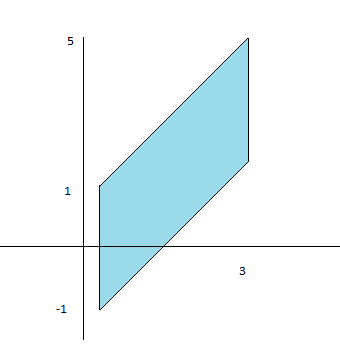# Match the graph of g(D) with the corresponding transformation of the rectangle D. ...

## Question:

Match the graph of g(D) with the corresponding transformation of the rectangle D.{eq}\begin{array}{l}{\text { A) transformation: } x=v \text { and } y=-u+2 v \text { with rectangle: } D \text { given by } 1 \leq u \leq 3 \text { and } 1 \leq v \leq 3} \\ {\text { B) transformation: } x=v \text { and } y=u-v \text { with rectangle: } D \text { given by } 2 \leq u \leq 3 \text { and } 1 \leq v \leq 3} \\ {\text { C) transformation: } x=u+v \text { and } y=-u+2 v \text { with rectangle: } D \text { given by } 1 \leq u \leq 3 \text { and }} {1 \leq v \leq 3} \\ {\text { D) none of these }}\end{array} {/eq}

## Double Integral:

We will find the correct transformation by checking the x and y points that are the coordinates of the parallelogram and then finding the values of u and v which will give the region.

To find the correct option we will find x and y in terms of u and v:

{eq}u=2x-y\\ v=x {/eq}

The points in the xy plane are (1,-1), (1,3), (3,5) and (1,1)

It will be transformed to u nad v plane where we will make the substituion:

{eq}x=v\\ y=-u+2v\\ 1\leq u\leq 3\\ 1\leq v\leq 3 {/eq}

Hence option a is correct